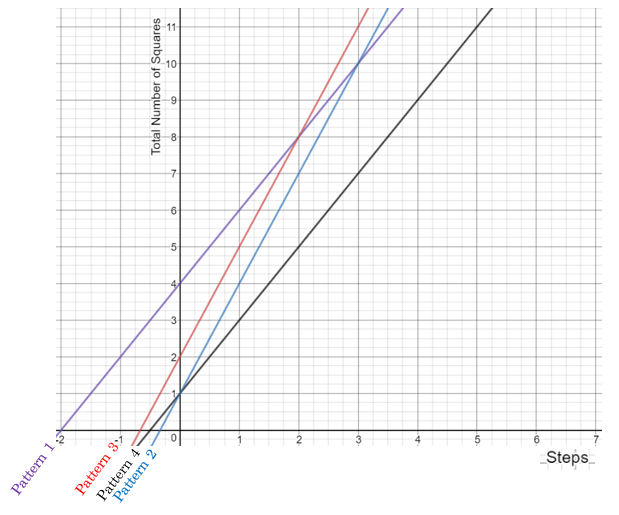# LINR 1 | Lesson 1 | Making Connections (Graphed Lines)

## Graphed Lines

Points from the four equation patterns are graphed (and connected) to form lines on one coordinate plane using Desmos.com as shown below.Describe patterns in the graphed lines that are similar.

• The graphed lines representing Patterns 1 and 4 have the same growth rate or slope of 2.
• The graphed lines representing Patterns 2 and 3 are parallel. Graphs with the same slope are parallel.
• The graphed lines representing Patterns 2 and 4 have the same $$y$$-intercept of $$(0,1)$$.
1. Write the equation for any two lines not shown above that are parallel. Name the slope.
2. Name the $$y$$-intercepts of the graphed lines representing Patterns 2 and 4.

Describe patterns in the graphed lines that are different.

• The graphed lines representing Patterns 1 and 3 have different slopes.
1. Write the equation for any two lines not shown above that have different slopes.
2. Name the lines that have different $$y$$-intercepts.

Check your solutions here.